Transaction Isolation Implementations in DBMS

In order to determine if a transaction is Serializable or not we need some thorough analysis of transactions. We cannot simply say any serial schedule transactions are conflicting or view Serializable. In order to determine their serializability and hence their isolation, we use precedence graph. This is a directed graph, from one transaction to another.  A directed node between any two transactions is drawn if there is any conflict between them.

Consider below case and the precedence graph for the same. Draw nodes for each transaction. Draw a directed line from T1 to T2 (T1 T2) if T2 reads the data x after T1 writes it, or if T2 writes x after T1 reads it.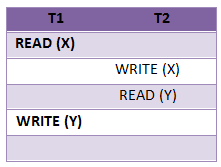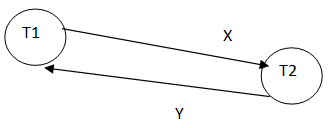A directed line between T1 and T2 is drawn in a schedule if we have combination of transactions in T1 and T2 as in below table.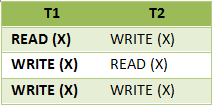A schedule is said to be conflict Serializable if and only if the precedence graph is acyclic. If the graph forms a cycle, then it cannot be conflict Serializable.

Consider below set of transaction and its precedence graph. Here it forms a cycle and hence it cannot be conflict Serializable.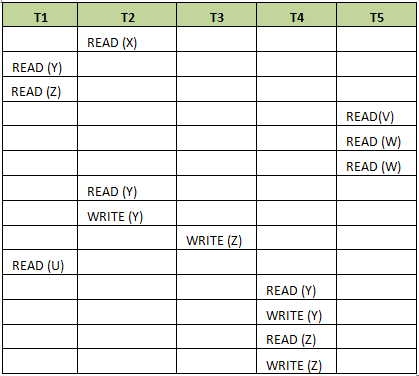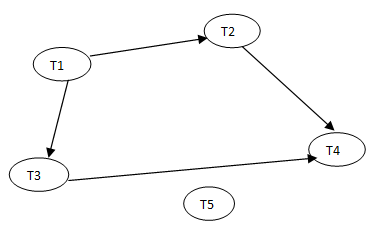For any schedule to be conflict Serializable, the precedence graph should be like T1 → T2 → T4 → T5 → T3 (order of transaction can be anything, but it should not form cycle). That means transactions can be serialized even if they have conflicting transactions, and can achieve isolation.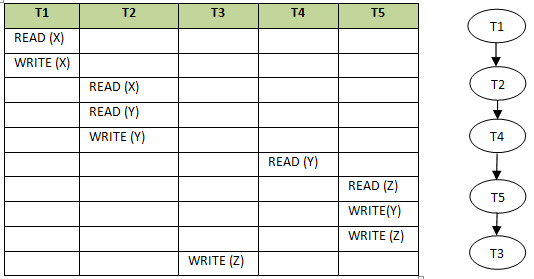Now let us see how to determine if a schedule is view Serializable or not. We cannot determine this by graph above, but it needs some analysis. Consider that we have three transactions – T1, T2 and T3. Now write all the combination of these three transactions.

Data Independence

T1, T2, T3
T1, T3, T2
T2, T1, T3
T2, T3, T1
T3, T1, T2
T3, T2, T1

According to view serializability rule stated above, if a transaction is reading the data in a schedule, it should read the data in other schedule too. Suppose T1 is reading the data. Then T1 should be the first transaction reading the data in both the schedules. Hence retain those transactions which read data first, T1 first. Below last combination of transactions are kept thing T2 is writing the data that T1 has read and T3 is reading some other data.

T1, T2, T3
T1, T3, T2
T3, T1, T2

According to second condition of view serializability, if a transaction reads the data written by another transaction in a schedule, then another schedule should also have a transaction which reads the data written by another same transaction. But in above case, T1 reads the initial data and should be the first transaction. T2 writes the data read by T1. T3 reads some other data. Hence we do not have case of transaction reading a data written by T2. Hence it cannot be view Serializable.

Suppose we passed second condition too. Now the third condition says, if one of the transactions writes the final data in a schedule, then same transaction should write the data in combination of transactions. In above combination, T2 writes the final data. But T3 reads some other data. Hence we do not have combination of at least 2 transactions which has T2 at the end and T1 at the beginning. Hence it cannot be view serializable.

Characteristics of a Database

Let consider another example below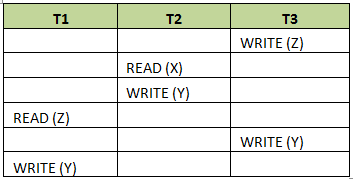This can even be re-written as W3 (Z), R2 (X), W2 (Y), R1 (Z), W3 (Y), W1 (Y).

• According first rule, transaction reading initial data should be kept at the beginning. Here T1 and T2 read the data. But T2 reads the initial data – since there is no writes before T2’s read. The read in T1 is after it is written by T3. Hence T1’s read cannot be considered as initial read. Hence, we should have T2 placed at the beginning or at least before other transaction reads it or before last transaction.
• According to second rule, transaction should read the data written by some other transaction. In above case, we see T3 writes X and is read by T1. Hence T1 should be placed after T3 i.e.; T3 → T1.
• As per third rule, the transactions writing the final results should be placed at the end. Here all the transactions write the result. If we observe, the final result is the result of Y or Z. But after writing Z, it is read by T1. Hence it is not final. Y is written by three transactions: T1, T2 or T3. As we discussed in first step, T2 reads the initial data and hence cannot be placed at the end. It will be placed at the beginning. T3 writes data X, and is red by T1. Hence it should be placed before T1. Now only left transaction is T1, which writes the final result Y, and can be kept at the end – may be after T2 or T3